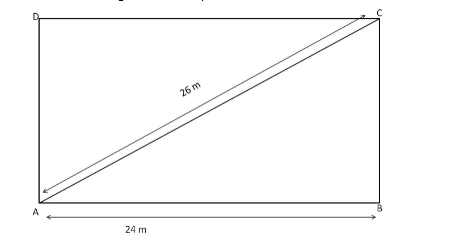# The longer side of a rectangular hall is 24 m and the length of its diagonal is 26 m.`
Question:

The longer side of a rectangular hall is 24 m and the length of its diagonal is 26 m. Find the area of the hall.

Solution:

Let the rectangle ABCD represent the hall.Using the Pythagorean theorem in the right-angled triangle ABC, we have:

Diagonal $^{2}=$ Length $^{2}+$ Breadth $^{2}$

$\Rightarrow$ Breadth $=\sqrt{\text { Diagonal }^{2}-\text { Length }^{2}}$

$=\sqrt{26^{2}-24^{2}}$

$=\sqrt{676-576}$

$=\sqrt{100}$

$=10 \mathrm{~m}$

$\therefore$ Area of the hall $=$ Length $\times$ Breadth $=24 \times 10=240 \mathrm{~m}^{2}$